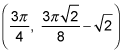##### Calculus: 1001 Practice Problems For Dummies (+ Free Online Practice)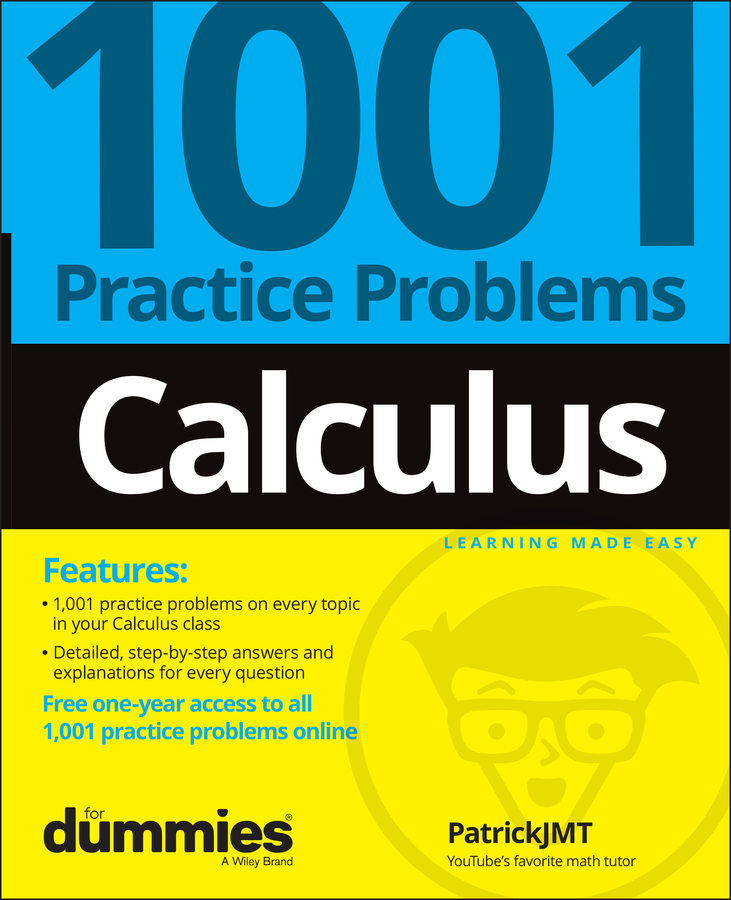You can use a derivative to locate the top of a "hill" and the bottom of a "valley," called local extrema, on just about any function. You simply set the derivative of the function equal to zero and solve for x.

A derivative is useful because it tells you the slope of a curve. This means that any problem involving anything about the slope or steepness of a curve is a derivative question.

## Practice questions

1. Use the first derivative to find the local extrema of f(x) = 6x2/3 – 4x + 1.

2. Find the local extrema of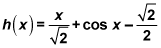in the interval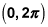with the first derivative test.

1. Using the first derivative of f(x) = 6x2/3 – 4x + 1, the local min is at (0, 1), and the local max is at (1, 3).

To find these local extrema, you start by finding the first derivative using the power rule.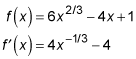Now you find the critical numbers of f. First, you set the derivative equal to zero and solve: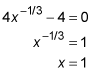Then, you need to determine the x values where the derivative is undefined.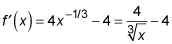Because the denominator is not allowed to equal zero,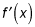is undefined at x = 0. Thus the critical numbers of f are 0 and 1. You can plot these critical numbers on a number line if it helps.

Plug a number from each of the three regions into the derivative.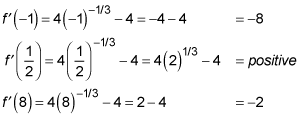Note how the numbers that were chosen for the first and third computations made the math easy. With the second computation, you can save a little time and skip the final calculation because all you care about is whether the result is positive or negative (this assumes that you know that the cube root of 2 is more than 1 — you'd better!).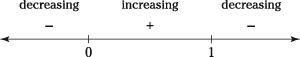Determine whether there's a local min or max or neither at each critical number.

f goes down to where x = 0 and then up, so there's a local min at x = 0, and f goes up to where x = 1 and then down, so there's a local max at x = 1.

Figure the y value of the two local extrema.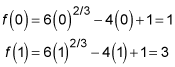Thus, there's a local min at (0, 1) and a local max at (1, 3). Check this answer by looking at a graph of f on your graphing calculator.

2. Using the first derivative test, forin the interval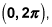the local max is at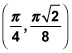and the local min is at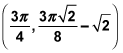And here's how the graph looks.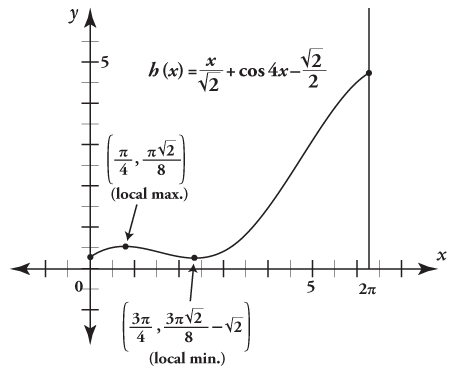Find the first derivative.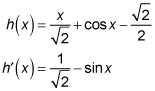Find the critical numbers of h.

Set the derivative equal to zero and solve: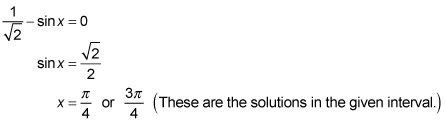Determine the x values where the derivative is undefined.

The derivative isn't undefined anywhere, so the critical numbers of h are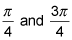Test numbers from each region on your number line.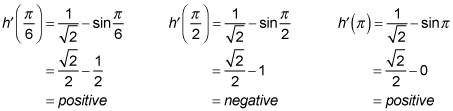Draw a sign graph.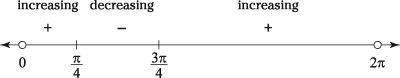Decide whether there's a local min, max, or neither at each of the two critical numbers.

Going from left to right along the function, you go up until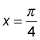and then down, so there's a local max atIt's vice versa for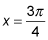so there's a local min there.

Compute the y values of these two extrema.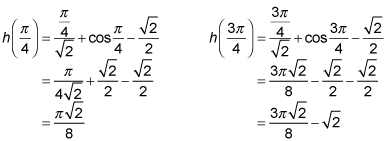So you have a max at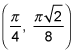and a min at